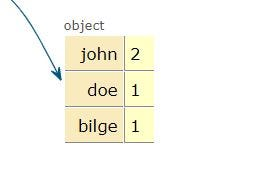# Easiest Way to Find the Most Frequent Element in ArrayPhoto by Caspar Camille Rubin on Unsplash

First of all, while browsing, I couldn't find the most effective and short solution to find the most frequent element in an array but I only found a lot of long solutions with for loop however the best way is not using nested for loop. Because in Big O Notation, it's a `O(n2)` complexity which means it is not effective.

The best way to find the most frequent element in an array is by using reduce function and creating a hashmap. And that's the whole code you need:

``````function getMostFrequent(arr) {
const hashmap = arr.reduce( (acc, val) => {
acc[val] = (acc[val] || 0 ) + 1
return acc
},{})
return Object.keys(hashmap).reduce((a, b) => hashmap[a] > hashmap[b] ? a : b)
}
``````

What I did was, creating a hashmap using reduce. If we have an array like `['john', 'doe', 'john', 'bilge']`, our hashmap will look like this:Because we create an object using acc in the first reduce function, notice the initial value of `acc` is {}.

Then we check for each value of the array: is this value already in `acc`?

If no, put a key-value pair in the object. ( first appearance of the element)

If yes, increment the value of it.

Once we have the hashmap with elements and their occurrence number in the array, then we just need to get the key with the biggest value.

For that, we simply find the biggest value in the array and return the key of it with the reduce function like this.

``````Object.keys(hashmap).reduce((a, b) => hashmap[a] > hashmap[b] ? a : b)
``````

Note: As you probably noticed, this will return only one key with the highest value, if you have two elements with the highest value and you want to return an array of it then you need to change the second reduce function.

In case you have not only one maximum in the array and you want to return an array of max values you can use:

``````return Object.keys(hashmap).filter(x => {
return hashmap[x] == Math.max.apply(null,
Object.values(hashmap))
})
``````

Notice this will return an array of the most frequent element, even if there is one. It will filter the elements which have not the max value and return it.

### Conclusion

And there we have it! How to find the most common element in an array. I hope you have found this useful!